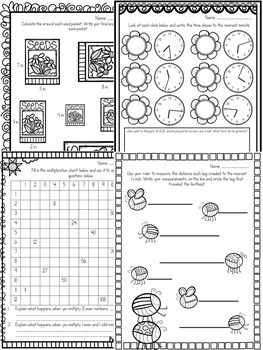# Spring NO PREP Math (3rd Grade)3rd
Subjects
Standards
Resource Type
Formats Included
• PDF
•Activity
Pages
50 pages
Easel Activity Included
This resource includes a ready-to-use interactive activity students can complete on any device. Easel by TpT is free to use! Learn more.

### Description

NO PREP Spring Math Packet (3rd Grade): This spring-themed math packet is full of plenty of practice opportunities for those sweet 3rd graders in your room! I have tried to pair each page to a common core standard. You can easily use these pages for centers, small group, morning work, and homework. I also included answer keys for every page. Here are the topics covered:

*Turning a multiplication array into a fact family & word problem

*Word Problems (addition, subtraction, multiplication, and division)

*2-Step Word Problems (require a mix of operations)

*Multiplication (solve and color; fill-in-the-blank)

*4 digit subtraction (with regrouping)

*Area (multiply to solve)

*Perimeter (calculate the perimeter of a "spring" banner")

*Extended Multiplication Facts (Example: 4 x 60)

*Spin an Extended Fact

*Fractions (comparing using <, >, and =)

*Fractions (partitioning a number line and placing the fraction on it)

*Spin and Graph (bar graph)

* Measuring to the nearest ¼ inch

*Rounding (nearest 10 and 100)

*Time (nearest minute + elapsed time bonus word problems)

*Spin a Fact Family

*Multiplication Chart Patterns

If you enjoyed this product, please be sure to check out:

Christmas NO PREP Math (3rd Grade)

Total Pages
50 pages
Included
Teaching Duration
N/A
Report this Resource to TpT
Reported resources will be reviewed by our team. Report this resource to let us know if this resource violates TpT’s content guidelines.

### Standards

to see state-specific standards (only available in the US).
Interpret products of whole numbers, e.g., interpret 5 Ã 7 as the total number of objects in 5 groups of 7 objects each. For example, describe a context in which a total number of objects can be expressed as 5 Ã 7.
Interpret whole-number quotients of whole numbers, e.g., interpret 56 Ã· 8 as the number of objects in each share when 56 objects are partitioned equally into 8 shares, or as a number of shares when 56 objects are partitioned into equal shares of 8 objects each. For example, describe a context in which a number of shares or a number of groups can be expressed as 56 Ã· 8.
Use multiplication and division within 100 to solve word problems in situations involving equal groups, arrays, and measurement quantities, e.g., by using drawings and equations with a symbol for the unknown number to represent the problem.
Determine the unknown whole number in a multiplication or division equation relating three whole numbers. For example, determine the unknown number that makes the equation true in each of the equations 8 Ã ? = 48, 5 = __ Ã· 3, 6 Ã 6 = ?.
Apply properties of operations as strategies to multiply and divide. Examples: If 6 Ã 4 = 24 is known, then 4 Ã 6 = 24 is also known. (Commutative property of multiplication.) 3 Ã 5 Ã 2 can be found by 3 Ã 5 = 15, then 15 Ã 2 = 30, or by 5 Ã 2 = 10, then 3 Ã 10 = 30. (Associative property of multiplication.) Knowing that 8 Ã 5 = 40 and 8 Ã 2 = 16, one can find 8 Ã 7 as 8 Ã (5 + 2) = (8 Ã 5) + (8 Ã 2) = 40 + 16 = 56. (Distributive property.)